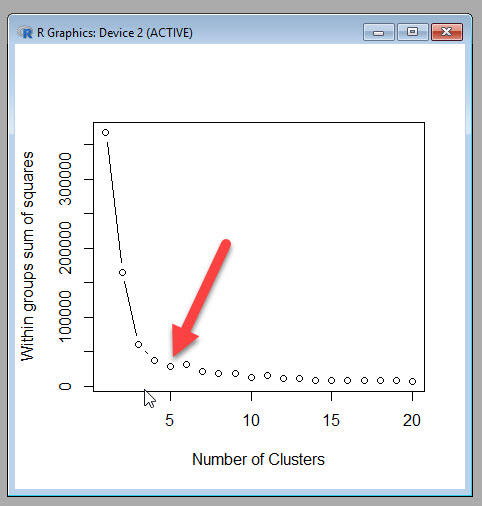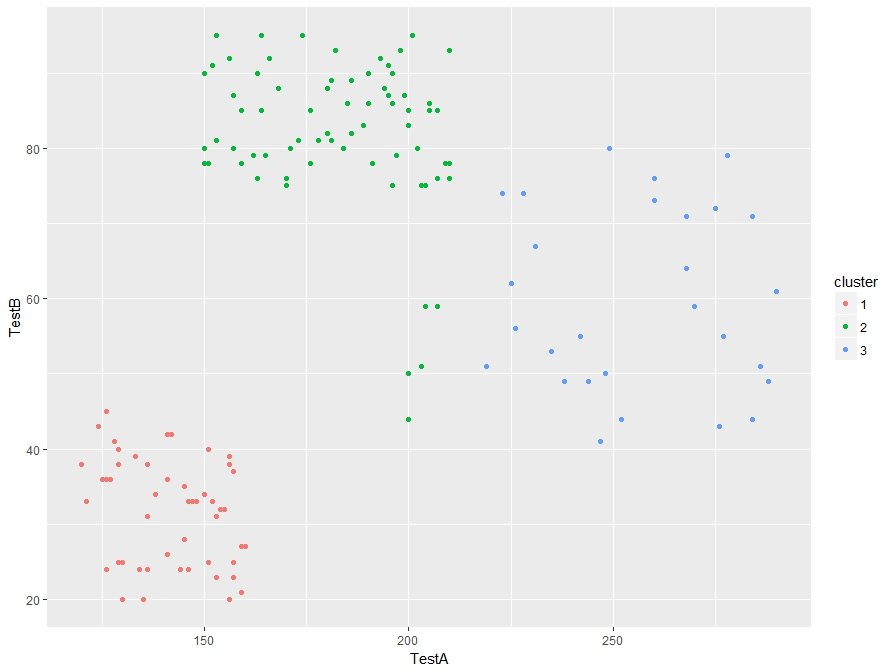# R: K-Means Clustering- Deciding how many clusters

In a previous lesson I showed you how to do a K-means cluster in R. You can visit that lesson here: R: K-Means Clustering.

Now in that lesson I choose 3 clusters. I did that because I was the one who made up the data, so I knew 3 clusters would work well. In the real world it doesn’t work that way. Choosing the right number of clusters is one of the trickier parts of performing a k-means cluster.

If you go over to Michael Grogan’s site, you will see he has a great method for figuring out how many clusters to choose. http://www.michaeljgrogan.com/k-means-clustering-example-stock-returns-dividends/

````wss <- (nrow(sample_stocks)-1)*sum(apply(sample_stocks,2,var))`
`for (i in 2:20) wss[i] <- sum(kmeans(sample_stocks,`
`centers=i)\$withinss)`
`plot(1:20, wss, type="b", xlab="Number of Clusters",`
`ylab="Within groups sum of squares")````

If you understand the code above, then great. That is a great solution for choosing the number of clusters. If, however, you are not 100% sure what is going on above, keep reading. I’ll walk you through it.

## K-Means Clustering

We need to start by getting a better understanding of what k-means clustering means. Consider this simplified explanation of clustering.

The way is works is each of the rows our data are placed into a vector.These vectors are then plotted out in space. Centroids (the yellow stars in the picture below) are chosen at random. The plotted vectors are then placed into clusters based on which centroid they are closest to.So how do you measure how good your clusters fit. (Do you need more clusters? Less clusters)? One popular metrics is the Within cluster sum of squares. R provides this as kmeans\$withinss. What this means is the distance the vectors in each cluster are from their respected centroid.

The goal is to get the is to get this number as small as possible. One approach to handling this is to run your kmeans clustering multiple times, raising the number of the clusters each time. Then you compare the withinss each time, stopping when the rate of improvement drops off. The goal is to find a low withinss while still keeping the number of clusters low.This is, in effect, what Michael Grogan has done above.

## Break down the code

Okay, now lets break down Mr. Grogan’s code and see what he is doing.

````wss <- (nrow(sample_stocks)-1)*sum(apply(sample_stocks,2,var))`
`for (i in 2:20) wss[i] <- sum(kmeans(sample_stocks,`
`centers=i)\$withinss)`
`plot(1:20, wss, type="b", xlab="Number of Clusters",`
`ylab="Within groups sum of squares")````

The first line of code is a little tricky. Let’s break it down.

`wss <- (nrow(sample_stocks)-1)*sum(apply(sample_stocks,2,var))`

sample_stocks – the data set

wss <-  – This simply assigns a value to a variable called wss

(nrow(sample_stocks)-1)  – the number of rows (nrow) in sample_stocks – 1. So if there are 100 rows in the data set, then this will return 99

sum(apply(sample_stocks,2,var)) – let’s break this down deeper and focus on the apply() function. apply() is kind of like a list comprehension in Python. Here is how the syntax works.

apply(data, (1=rows, 2=columns), function you are passing the data through)

So, let’s create a small array and play with this function. It makes more sense when you see it in action.

``` tt <- array(1:20, dim=c(10,2)) # create array with data 1 -20,
#10 rows, 2 columns
> tt
[,1] [,2]
[1,] 1 11
[2,] 2 12
[3,] 3 13
[4,] 4 14
[5,] 5 15
[6,] 6 16
[7,] 7 17
[8,] 8 18
[9,] 9 19
[10,] 10 20```

Now lets try running this through apply.

```> apply(tt, 2, mean)
 5.5 15.5```

Apply took the mean of each column. Had I used 1 as the second argument, it would have taken the mean of each row.

```> apply(tt, 1, mean)
 6 7 8 9 10 11 12 13 14 15```

Also, keep in mine, you can create your own functions to be used in apply

```apply(tt,2, function(x) x+5)
[,1] [,2]
[1,] 6 16
[2,] 7 17
[3,] 8 18
[4,] 9 19
[5,] 10 20
[6,] 11 21
[7,] 12 22
[8,] 13 23
[9,] 14 24
[10,] 15 25
```

So, what is Mr. Grogan’s doing with his apply function? apply(sample_stocks,2,var) – He is taking the variance of each column his data set.

``` apply(tt,2,var)
 9.166667 9.166667```

And by summing it: sum(apply(sample_stocks,2,var)) – he is simply adding the two values together.

``` sum(apply(tt,2,var))
 18.33333```

So, the entire first line of code using our data is:

wss <- (nrow(tt)-1)*sum(apply(tt,2,var))

wss <- (10-1) * (18.333)

```wss <- (nrow(tt)-1)*sum(apply(tt,2,var))
> wss
 165```

What this number is effectively is the within sum of squares for a data set that has only one cluster

### Next section of code

Next we will tackle the next two lines of code.

````for (i in 2:20) wss[i] <- sum(kmeans(sample_stocks,`
`centers=i)\$withinss)````

The first part is a for loop and should be simple enough. Note he doesn’t use {} to denote the inside of his loop. You can do this when your for loop is a single line, but I am going to use the {}’s anyway, as I think it makes the code a bit neater.

for (i in 2:20)  — a for loop iterating from 2 -20

for (i in 2:20) {

wss[i] <- }  – we are going to assign more values to the vector wss starting at 2 and working our way down to 20.

Remember, a single value variable in R is actually a single value vector.

```c <- 5
> c
 5
> c <- 7
> c
 5 7```

Okay, so now to the trickier code. sum(kmeans(sample_stocks, centers = i)\$withinss)

What he is doing is running a kmeans cluster for the data one time each for each value of centers (number of centroids we want) from 2 to 20 and reading the \$withinss from each run. Finally it sums all the withinss up (you will have 1 withinss for every cluster you create – number of centers)

### Plot the results

The last part of the code is plotting the results

````plot(1:20, wss, type="b", xlab="Number of Clusters",`
`ylab="Within groups sum of squares")````

plot (x, y, type= type of graph, xlab = label for x axis, ylab= label for y axis

## Let’s try it with our data

``` myData <- read.csv('cluster.csv')
StudentId TestA TestB
1 2355645.1 134 24
2 8718152.6 155 32
3 8303333.6 130 25
4 6352972.5 185 86
5 3381543.2 153 95
6 817332.4 153 81
> myData <- myData [,2:3] # get rid of StudentId column
TestA TestB
1 134 24
2 155 32
3 130 25
4 185 86
5 153 95
6 153 81```

Now lets feed this through Mr. Grogan’s code

````wss <- (nrow(myData)-1)*sum(apply(myData,2,var))`
```for (i in 2:20) {
wss[i] <- sum(kmeans(myData,```
```          centers=i)\$withinss)
```         }
`plot(1:20, wss, type="b", xlab="Number of Clusters",`
`ylab="Within groups sum of squares")````

Here is our output ( a scree plot for you math junkies out there)Now Mr. Grogan’s plot a nice dramatic drop off, which is unfortunately not how most real world data I have seen works. I am going to chose 5 as my cut off point, because while the withinss does continue to decrease, it doesn’t seem to do so at a rate great enough to accept the added complexity of more clusters.

If you want to see how 5 clusters looks next to the three I had originally created, you can run the following code.

```myCluster <- kmeans(myData,5, nstart = 20)
myData\$cluster <- as.factor(myCluster\$cluster)
ggplot(myData, aes(TestA, TestB, color = cluster))
+ geom_point()```

5 Clusters3 ClustersI see some improvement in the 5 cluster model. So Michael Grogan’s trick for finding the number of clusters works.

## 4 thoughts on “R: K-Means Clustering- Deciding how many clusters”

1.Anonymous

Superbly explained! Thankyou

2.Anonymous

excellent

3.Anonymous

really good explanation